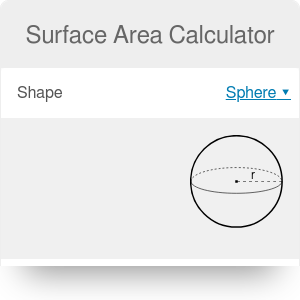Shape
sphere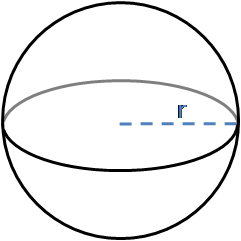in
Surface area
Surface area
in²

# Surface Area Calculator

By Bogna Haponiuk and Hanna Pamuła, PhD candidate

This surface area calculator helps you find the area of the most common three-dimensional solids. If you ever wondered how to find surface area or what is the lateral surface area, this calculator is here to help you. In this article, you can find the formulas for surface area of a sphere, cube, cylinder, cone, pyramid and rectangular/triangular prism. We will also explain how to calculate the surface area of a sphere as an example.

## What is a surface area? Surface area definition

Surface area is a total area that the surface of the object occupies. In other words, it's the total area of the surface of a 3D object.

Sometimes the surface area may be split into a sum of base area(s) and lateral surface area. The lateral surface is the area of all the sides of the object, excluding the area of its base and top. This division is used for shapes which have an obvious distinction between base and the other part - e.g., for a cylinder, cone, a pyramid or a triangular prism. It's rarely applied for solids for which we are not sure which faces should be treated as bases (like in a cube or box), and it's not used for smooth surfaces such as a sphere.

## Formula for the surface area of...

Our surface area calculator can find the surface area of seven different solids. The formula depends on the type of the solid.

• Surface area of a sphere: `A = 4πr²`, where r stands for the radius of the sphere.
• Surface area of a cube: `A = 6a²`, where a is the side length.
• Surface area of a cylinder: `A = 2πr² + 2πrh`, where r is the radius and h is the height of the cylinder.
• Surface area of a cone: `A = πr² + πr√(r² + h²)`, where r is the radius and h is the height of the cone.
• Surface area of a rectangular prism (box): `A = 2(ab + bc + ac)`, where a, b and c are the lengths of three sides of the cuboid.
• Surface area of a triangular prism: `A = 0.5 * √((a + b + c) * (-a + b + c) * (a - b + c) * (a + b - c)) + h * (a + b + c)`, where a, b and c are the lengths of three sides of the triangular prism base and h is a height (length) of the prism.
• Surface area of a pyramid: `A = l * √(l² + 4 * h²) + l²`, where l is a side length of the square base and h is a height of a pyramid.

But where do those formulas come from? How to find the surface area of the basic 3D shapes? Keep reading and you'll find out!

## Surface area of a sphereTo calculate the surface area of a sphere, all you need to know is a sphere radius - or its diameter.

• `A = 4 * π * r²` where `r` is the radius

As we know that diameter of a sphere is equal to two radii `d = 2r`, we can transform the equation into another form:

• `A = 4 * π * (d / 2)² = π * d²` where `d` is the sphere diameter

The derivation of this surface area formula requires integration. If you're curious, check out this proof.

## Surface area of a cylinder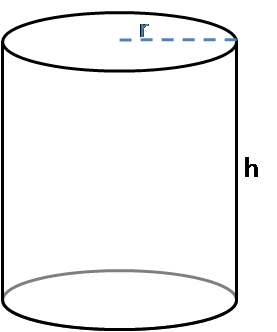To find out what's the surface area of a cylinder, you should have two values given: radius (or diameter) of a base and the height of the cylinder. The general equation is as usual - base area times height. In our case, a circle is a base.

• `A = 2πr² + 2πrh`

Where does the formula come from? You can write the equation for the surface area of a cylinder as:

• `A = A(lateral) + 2 * A(base)`

It's easy to find a base area - we remember the well-known formula for the area of a circle: `A(base) = π * r²`. But what's the shape of the lateral surface area? Try to imagine that we "unfold" it. Do you recognize it? It's a rectangle! One side length is a cylinder height and second one is the unfolded circle circumference.

• `A(base) = π * r²`
• `A(lateral) = h * (2 * π * r)`

## Surface area of a cone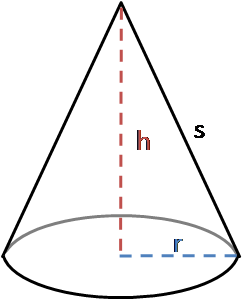The surface area of a cone may also be split into two parts:

• `A = A(lateral) + A(base)`, as we have only one base, in contrast to a cylinder.

The base is again the area of a circle `A(base) = π * r²`, but the lateral surface area origins may be not so obvious:

• `A(lateral) = π * r * √(r² + h²)`

Let's have a look at this step by step derivation:

1. Roll the lateral surface out flat. It's a circular sector, which is the part of a circle with radius s (s is the slant height of the cone).
2. For the circle with radius s, the circumference is equal to `2 * π * s`. The arc length of the sector is equal to `2 * π * r`.
3. The area of a sector - which is our lateral surface of a cone - is given by the formula:
• `A(lateral) = (s * (arc length)) / 2 = (s * 2 * π * r) / 2 = π * r * s`

The formula can be obtained from proportion: ratio of the areas of the shapes is the same as ratio of the arc length to the circumference:

• `(sector area) / (large circle area) = (arc length) / (large circle circumference)` so
• `(sector area) / (π * s²) = (2 * π * r) / (2 * π * s)`
• `(sector area) = (π * s²) * (2 * π * r) / (2 * π * s)`
• `A(lateral) = π * r * s`
1. Usually we don't have the `s` value given but `h`, which is the height of the cone. But that's not a problem at all! We can easily transform the formula using Pythagorean theorem:
• `r² + h²= s²` so taking the square root we got `s = √(r² + h²)`

Thus, the lateral surface area formula looks as follows:

• `A(lateral) = π * r * √(r² + h²)`
1. Finally, add the areas of the base and the lateral part to find the final formula for surface area of a cone:
• `A = A(lateral) + A(base) = π * r * s + π * r²` given `r` and `s` or
• `A = π * r * √(r² + h²) + π * r²` given `r` and `h`.

## Surface area of a cube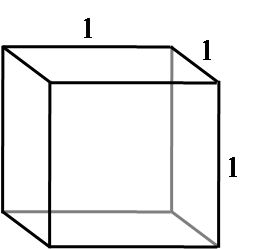The surface area of a cube is the easiest you can imagine: each of the sides is a square! As every cube has six identical square faces, the surface area equals:

• `A = 6 * (side area)`

As the area of a square is the product of the length of its sides, the final formula for the surface area of a cube is:

• `A = 6 * l²`, where `l` is a square side

## Surface area of a pyramid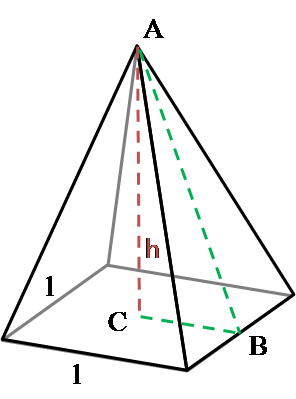A pyramid is a 3D solid with a polygonal base and triangular lateral faces. When you hear a pyramid, it's usually assumed to be a regular square pyramid. Regular means that it has a regular polygon base and is a right pyramid (apex directly above the centroid of its base) and square - that it has this shape as a base. That's the option which we used as a pyramid in this surface area calculator.

The formula for surface area of a pyramid is:

• `A = l * √(l² + 4 * h²) + l²` where `l` is a base side and `h` is a height of a pyramid

Again, we can split the equation into:

• `A = A(base) + A(lateral) = A(base) + 4 * A(lateral face)`

The base is in the shape of square, so `A(base) = l²`. To calculate the lateral surface area, let's start with the area of one triangular face:

1. To find the height of the triangle, we will need the formula for hypotenuse again:
• `c = √(a² + b²)`
1. Calculate the triangle ABC hypotenuse (which is at the same time the height of the triangular face):
• `c = √(h² + (l/2)²) = √(h² + l²/4)`
1. The area of a triangle (in our case it's isosceles triangle) may be calculated as:
• `A = height * base / 2` so
• `A(lateral face) = √(h² + l²/4) * l / 2`
1. So the final formula for the surface area of a pyramid is:
• `A = l² + 4 * √(h² + l²/4) * l / 2 = l² + 2 * l * √(h² + l²/4)`
• `A = l² + l * √(4 * h² + l²)`

## Surface area of a rectangular prism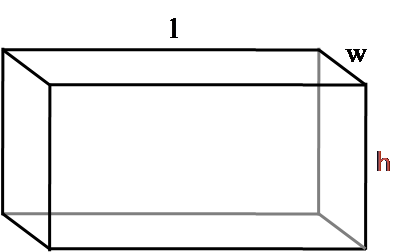To calculate the surface area of a rectangular prism, all you need to do is to calculate the areas of the rectangular sides:

• `A = 2 * (A1 + A2 + A3)`

where:

• `A1 = l * w`
• `A2 = w * h`
• `A3 = l * h`

Thus, the final formula is:

• `A = 2 * (l * w + w * h + l * h)`

## Surface area of a triangular prism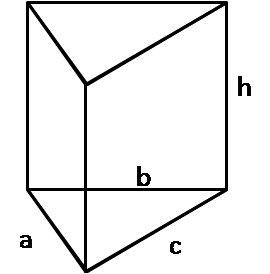To understand where the formula for the surface area of a triangular prism comes from, let's have a look at this derivation:

1. The lateral surface area part is easy to calculate in this case. As you can see from the picture, it consists of three rectangles, with one side length in common:
• `A(lateral) = a * h + b * h + c * h = h * (a + b + c)`

which we can also write in short as:

• `A(lateral) = h * P`, where `P` is a perimeter of a base triangle
1. Then, find the area of a triangular base. You can do it in many ways, depending on what's given. In our calculator, we've implemented the calculation on the basis of the Heron's formula - it's used when you have three triangle sides given(SSS).

`A(base) = 0.25 * √((a + b + c) * (-a + b + c) * (a - b + c) * (a + b - c)))`

1. The final formula for a surface area of a triangular prism:
• `A = A(lateral) + 2 * A(base)`
• `A = h * (a + b + c) + 0.5 * √((a + b + c) * (-a + b + c) * (a - b + c) * (a + b - c))`

## Body surface area

You can calculate the surface of any solid, for example, your body - it doesn't need to be the simple shape from geometry! If you're curious what's the external surface area of a human body, check out this body surface area calculator.

## How to calculate the surface area of a sphere?

If you want to find the surface area of a sphere, you need to follow these steps:

1. Determine the radius of the sphere. We can assume a radius of 10 cm.
2. Input this value into the formula `A = 4πr²`.
3. Calculate the result: `A = 4π * 10² = 1256 cm²`.
4. You can also use this surface area calculator to find the radius of the sphere, knowing its area.

## Other considerations

The units of surface area are always square units of length. For example, you can express it in cm², in², ft², m², but also in acres and hectares.

If you want to find the volume of any of these solids, use our volume calculator to do it.

Bogna Haponiuk and Hanna Pamuła, PhD candidate

## Get the widget!

Surface Area Calculator can be embedded on your website to enrich the content you wrote and make it easier for your visitors to understand your message.

It is free, awesome and will keep people coming back!